Electron. J. Diff. Eqns., Vol. 2004(2004), No. 98, pp. 1-28.

### Structural stability of polynomial second order differential equations with periodic coefficients Adolfo W. Guzman

Abstract:
This work characterizes the structurally stable second order differential equations of the form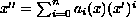where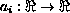are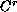periodic functions. These equations have naturally the cylinder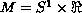as the phase space and are associated to the vector fields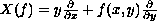, where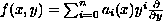.
We apply a compactification to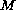as well as to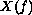to study the behavior at infinity. For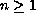, we define a set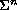ofthat is open and dense and characterizes the class of structural differential equations as above.

Submitted April 29, 2004. Published August 9, 2004.
Math Subject Classifications: 37C20.
Key Words: Singularity at infinity; compactification; structural stability; second order differential equation.

Show me the PDF file (499K), TEX file, and other files for this article.Adolfo W. Guzman Departamento de Matematica Universidade Federal de Vicosa Campus Universitario CEP 36571-000. Vicosa - MG. Brasil email: guzman@ime.usp.br   guzman@ufv.br